## Selina Concise Mathematics Class 10 ICSE Solutions Revision Paper 2

Section A (40 Marks)
(Answer all questions from this Section)

Question 1.
(a) In what ratio does the line x – y – 2 = 0 divide the line segment joining the points (3, -1) and (8, 9)? Also, find co-ordinates of the point of intersection.
Solution:
The given two points are A (3, -1) and B (8, 9)
∴ Slope of line = $$\frac{y_{2}-y_{1}}{x_{2}-x_{1}}=\frac{9+1}{8-3}=\frac{10}{5}=2$$
and equation of AB
y – 9 = 2(x – 8) ⇒ y – 9 = 2x – 16
2x – y = 7 …….(i)
and line given x – y – 2 = 0 ⇒ x – y = 2 …..(ii)
Subtracting (ii) from (i/), we get x = 5
and y = x – 2 = 5 – 2 = 3
∴ Co-ordinates of point of intersection = (5, 3)
Now let ratio = m : n
$$5=\frac{m x_{2}+m x_{1}}{m+n}=\frac{8 m+3 n}{m+n}$$
⇒ 8m + 3n = 5m + 5n ⇒ 8m – 5m = 5n – 3n ⇒ 3m = 2n
⇒ $$\frac{m}{n}=\frac{2}{3}$$
∴ Ratio = 2 : 3

(b) Find :
(i) the fourth proportional to 2a, 3b and 4c.
(ii) the mean proportional to x – y and (x – y)3.
(iii) the third proportional to a + b and $$\sqrt{a^{2}-b^{2}}$$
Solution:
(i) Fourth proportional of 2a, 3b, 4c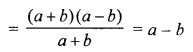(c) Using remainder theorem, factorise : x3 + 7x2 – 21x – 27 completely and then solve x3 + 7x2 – 21x – 27 = 0
Solution:
Let f(x) = x3 + 7x2 – 21x – 27
f(3) = (3)3 + 7(3)2 – 21 × 3 – 27
= 27 + 63 – 63 – 27 = 0
∴ x – 3 is a factor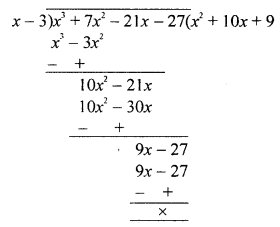Dividing f(x) by x – 3,
f(x) = (x – 3) (x2 + 10x + 9)
= (x – 3)[x2 + x + 9x + 9]
= (x – 3)[x(x + 1) + 9(x + 1)]
= (x — 3) (x + 1) (x + 9)
Now, x2 + 7x2 – 21x – 27 = 0
⇒ (x – 3) (x + 1) (x + 9) = 0
Either x – 3 = 0, then x = 3
or x + 1 = 0, then x = -1
or x + 9 = 0, then x = -9
Hence x = 3, -1, -9

Question 2.
(a) Find the 99th term of the series :Solution:(b) Metallic spheres of diameters 12 cm, 16 cm and 20 cm respectively, are melted to form a single solid sphere. Find the radius of the resulting sphere.
Solution:
Diameter of first sphere = 12 cm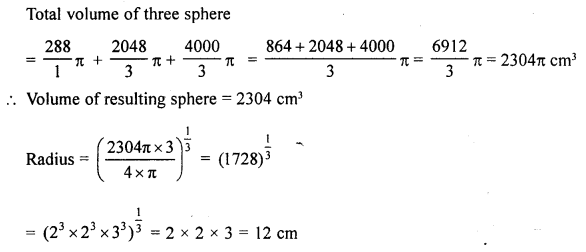(c) If A = $$\left[\begin{array}{ll}{0} & {4} \\ {1} & {0}\end{array}\right], \mathbf{B}=\left[\begin{array}{cc}{-2} & {0} \\ {3} & {-2}\end{array}\right] \text { and } \mathbf{C}=\left[\begin{array}{cc}{-1} & {-2} \\ {2} & {\mathbf{0}}\end{array}\right]$$ show that : (B – C) A = BA – CA.
Solution: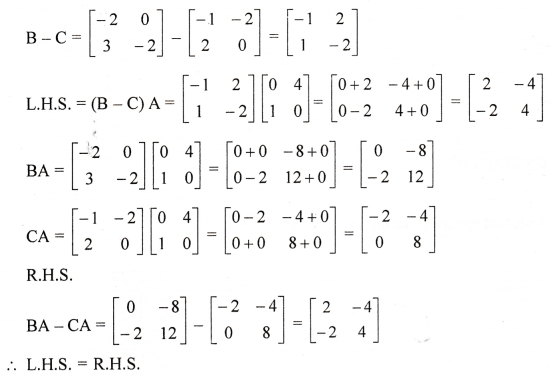Question 3.
(a) In the given figure, AB is diameter of the circle with centre O. AQ, BP and PRQ are tangents. Prove that OP and OQ are perpendicular to each other.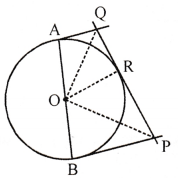Solution:
In the given figure,
AB is diameter of the cirlce with centre O. AQ, BP and PRQ are tangents to the circle.
To prove : OP ⊥ OQ
Proof: ∵ QA and QR the tangents to the circle
∴ QA=QR
∴ QO is the bisectors of ∠AOR
Similarly, PR and PB are tangents
∴ PR = PB
∴ PO is the bisector of ∠ROB
But ∠AOR + ∠ROB = 180° (Linear pair)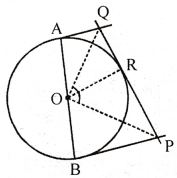⇒ $$\frac{1}{2}$$ ∠AOR + $$\frac{1}{2}$$ ∠ROB = 90°
⇒ ∠QOR + ∠ROP = 90° ⇒ QOP = 90°
∴ OP ⊥ OQ

(b) In the inequation $$2+\frac{3 x-1}{5} \leq \frac{2 x-1}{4}+3$$, write the greatest value of x, when :
(i) x is a natural number.
(ii) x is a real number.
Solution: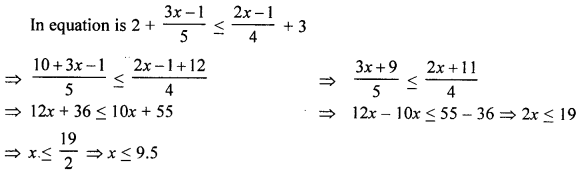(i) If x is a natural number, then greatest value is 9
(ii) If x is a real number, then greatest value of x is 9.5

(c) Find the missing frequencies in the following distribution table. It is given that the mean of these distributions is 56 and their total is 90 i.e., Σf = 90.

 Class interval 0-20 20-40 40-60 60-80 80-100 100-120 Frequency 16 f1 25 f2 12 10

Solution:
Mean = 56, total frequency i. e., Σf = 90

 Class interval Mid value (x) Frequency (f) f.x. 0-20 10 16 160 20-40 30 f1 30f1 40-60 50 25 1200 60-80 70 f2 70f2 80-100 90 12 1080 100-120 110 10 1100 Total 90 3590 + 30f1 + 70f2and f1 = 27 – f2 = 27 – 16 = 11
Hence, f1 = 11, f2 = 16

Question 4.
(a) Ramesh deposits ₹ 2,400 per month in a recurring deposit scheme of a bank for one year. If he gets ₹ 1,248 as interest at the time of maturity, find the rate of interest. Also, find the maturity value of this deposit.
Solution:
Deposit per month (P) = ₹ 2400
Period (n) = 1 year = 12 months
interest = ₹ 1248
Let rate % = r%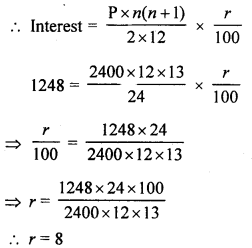Rate of 8%
Maturity value = Principal deposited + Interest = ₹ 2400 × 12 + ₹ 1248
= ₹ 28800 + ₹ 1248 = ₹ 30,048

(b) A (-2, 4) and B (-4, 2) are reflected in the .y-axis. If A’ and B’ are images of A and B respectively.
(i) Find the co-ordinates of A’ and B’.
(ii) Assign a special name to quadrilateral AA’B’B.
(iii) State whether AB’ = BA’.
Solution:
(i) A (-2, 4) and B (-4, 2) is reflected in y-axis
(ii) Image of A is A’ (2, 4)
and image of B is B’ (4, 2)
(iii) By joining A, B, A’, B’ ; ,
We get a trapezium in which AB’ = BA’

(c) A lot consists of 144 ball pens of which 20 are defective. A customer will buy a pen only if it is not defective. The shopkeeper draws one pen at random and gives to the customer. What is the probability that :
(i) the customer will buy it ?
(ii) the customer will not buy it ?
Solution:
Number of total ball pens = 144
No. of defective pens = 20
∴ Number of non-defective = 144-20 = 124
(i) One pen is drawn, then probability of non-defected pen = $$\frac{124}{144}=\frac{31}{36}$$
(ii) Probability of defective pen = $$\frac{20}{144}=\frac{5}{36}$$

Section B (40 Marks)
(Answer any four questions from this Section)

Question 5.
(a) In the given figure, tangents PQ and PR are drawn to a circle such that angle RPQ = 30°. A chord RS is drawn parallel to the tangent PQ. Find the angle RQS.Solution:
(a) In the given figure,
PQ and PR are the tangents to the circle
Such that ∠RPQ = 30°
A chord RS || PQ
RQ and SQ are joined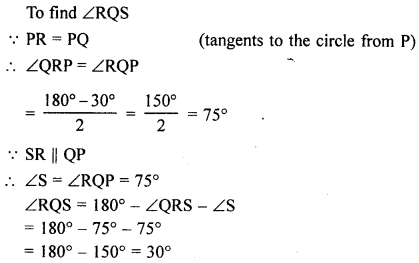(b) Prove that: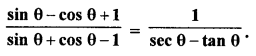Solution: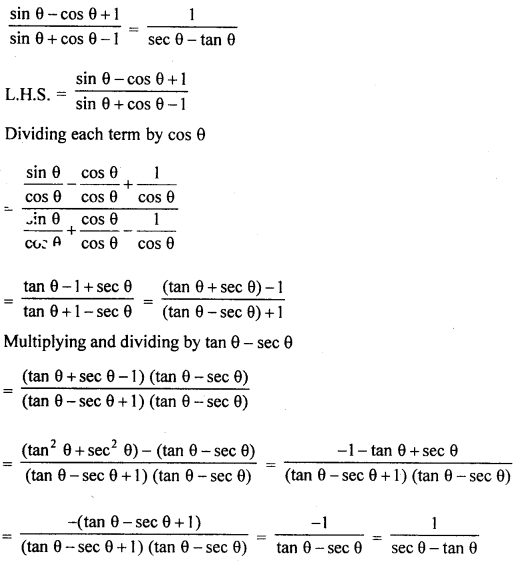= R.H.S

(c) The arithmetic mean between a and b is twice the geometric mean between a and b.
Prove that: $$\frac{a}{b}=7+4 \sqrt{3} \text { or } 7-4 \sqrt{3}$$.
Solution:
Given A.M. between a and b is twice the G.M. between a and b.
i. e., A.M. between a and b = 2 × G.M. between a and b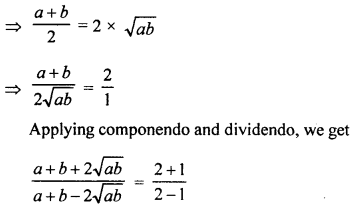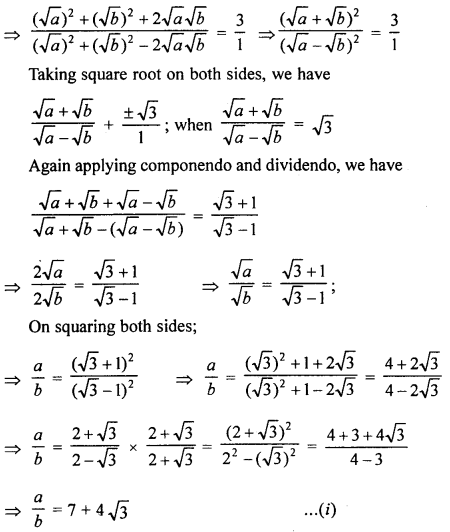Question 6.
(a) The marked price of an electronic device is ₹ 9,200 and the sales-tax levied on it is 8%. A customer asks the trader to give this electronic device for ₹ 9,000 or less as he has only ₹ 9,000 with him. The trader charged ₹ 8,856 only including sales-tax. Find the discount given by the trader.
Solution:
M.P. of an electric device = ₹ 9200
Rate of sale tax = 8%
S.P. of the device (including S.T.) = ₹ 8856
Price without S.T. = $$\frac{8856 \times 100}{10+8}$$ = ₹ 8200
∴ Discount = ₹ 9200 – ₹ 8200 = ₹ 1000

(b) The diagrams, given below, represent two inequations A and B on real number line.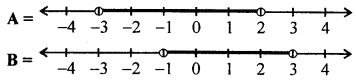(i) Write down A and B in set builder notations.
(ii) Represent A ∩ B and A’ ∩ B on two different number lines.
Solution:
Equations of A and B on number line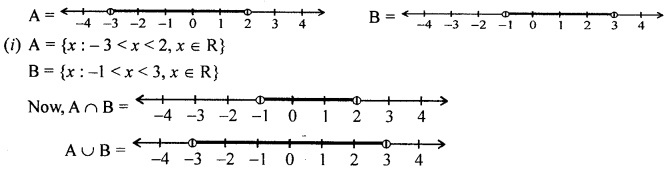(c) Solve for matrices A and B, where 2 A + B = $$=\left[\begin{array}{cc}{3} & {-4} \\ {2} & {7}\end{array}\right]$$ and A – 2B = $$\left[\begin{array}{ll}{4} & {3} \\ {1} & {1}\end{array}\right]$$
Solution: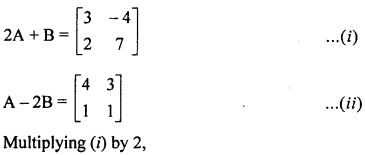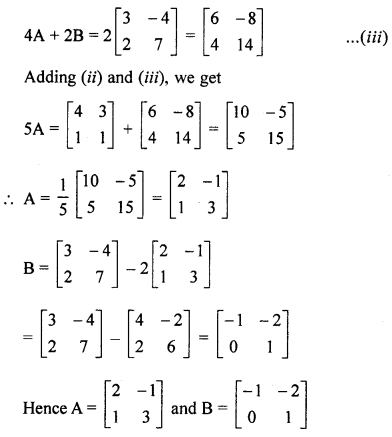Question 7.
(a) Solve the equation 3x2 – x – 1 = 0 and give your answer correct to two decimal places.
Solution:
3x2 – x – 7 = 0
Here, a = 3, b = -1, c = -7
D = b2 – 4ac = (-1 )2 – 4 × 3 × (-7)
= 1 + 84 = 85(b) Two identical solid cones each of base radius 3 cm with vertical height 5 cm and one more solid cone of base radius 2 cm with vertical height 4.5 cm are jointly melted and recasted into a solid sphere. Find : (i) the radius, (ii) curved surface area of the sphere.
Solution:
Radius of each indentical cone (h1) = 3 cm
and height (h1) = 5 cm
Radius of third cone (r2) = 2 cm
and vertical height (h1) = 4.5 cm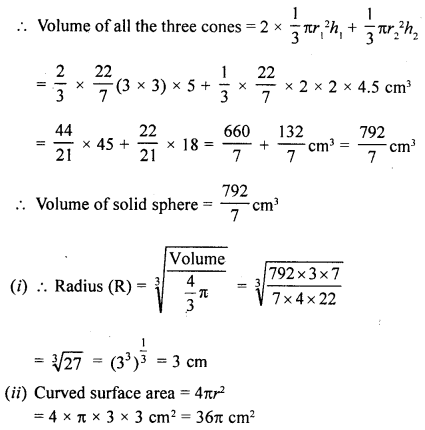(c) The angle of elevation of a cloud from a point h metres above the surface of a lake is 0 and the angle of depression of its reflection in the lake is ϕ. Prove that the height of the cloud above the lake surface is : $$h\left(\frac{\tan \phi+\tan \theta}{\tan \phi-\tan \theta}\right)$$.
Solution:
Let AM be the lake P is the cloud and P’ is its reflection in the lake.
Let the height of the cloud
MP = x m and its reflection MP’ = x
Now in right ∆PBC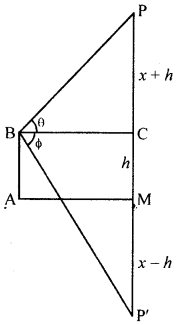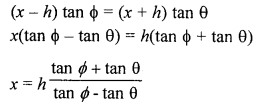Question 8.
(a) A man desires to have an annual income of ₹ 36,000 from 18% ₹ 125 shares available at a premium of 20%. How much should he invest?
Solution:
Annual income from 125 shows = ₹ 36000
Rate of dividend = 18%
Market rate = ₹ 100 + 20 = ₹ 120
∴ Investment = ₹ $$\frac{36000 \times 120}{18}$$ = ₹ 240000

(b) As shown in the given figure; from an external point P, a tangent PT and a line segment PAB are draw to a circle with centre O. ON is perpendicular on the chord AB. Prove that:
(i) PA.PB = PN2 – AN2
(ii) PN2 – AN2 = OP2 – OT2
(iii) PA.PB = PT2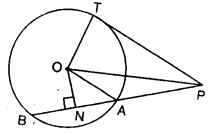Solution:
In the given figure,
PT is a tangent to the circle with centre O
OAB is a secant intersecting the circle at A and B
ON ⊥ AB
To prove :
(i) PA.PB = PN2 – AN2
(ii) PN2 – AN2 = OP2 – OT2
(iii) PA.PB = PT2
Construction : Join OT, OP and OA.
Proof:
(i) PA.PB = (PN – AN) (PN + BN)
= (PN – AN) (PN + AN) (∵ N is the mid point of AB)
= (PN)2 – (AN)2(ii) PN2 – AN2 = (OP2 – ON2) – (OA2 – ON2) (In right ∆ONP and ∆ONA)
= OP2 – ON2 – OA2 + ON2
= OP2 – OA2
= OP2 – OT2 (∵ OA = OT radii of the same circel)(iii) PA.PB = PN2 – AN2 [Proved in (i)]
= OP2 – OT2 [Proved in (ii)]
= PT2

(c) If x =$$\frac{\sqrt{2 a+1}+\sqrt{2 a-1}}{\sqrt{2 a+1}-\sqrt{2 a-1}}$$, prove that : x2 – 4ax + 1=0.
Solution: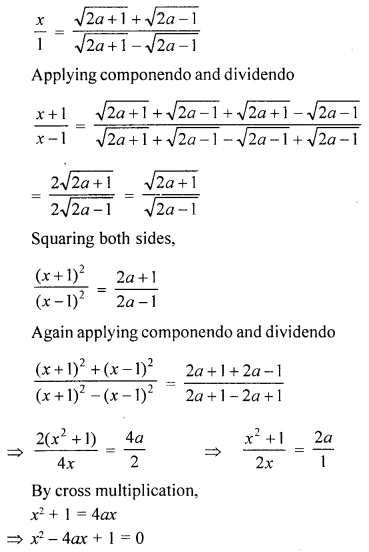Question 9.
(a) Find the co-ordinates of the point Q on x-axis which lies on the perpendicular bisector of the line segment joining the points A (-5, -2) and B (4, -2). Name the type of the triangle QAB.
Solution:
(a) Slope of line joining the points A (-5, -2) and B (4, -2) = $$\frac{y_{2}-y_{1}}{x_{2}-x_{1}}=\frac{-2+2}{4+5}=\frac{0}{9}=0$$
∴ AB || x-axis
Let co-ordinates of Q be (x, 0) and equation of line AB is y = -2
LM is perpendicular bisector of AB, which intersects x-axis at Q
Co-ordinates of Q are $$\left(\frac{-1}{2}, 0\right)$$
∵ Q lies on the right bisector of AB∴ QA = QB
∴ ∆QAB is an isosceles triangle.

(b) Two pipes running together can fill an empty cistern in $$11 \frac{1}{9}$$ minutes. If one pipe takes 5 minutes more than the other to fill the same cister, find the time in which each pipe would fill the cister.
Solution:
Work of two pipes for filling in 1 minute = $$\frac{9}{100}$$
Let one pipe takes x minutes
then second pipe will take (x + 5) minutes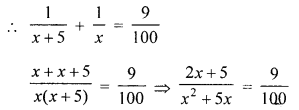⇒ 9x2 + 45x = 200x + 500
⇒ 9x2 + 45x – 200x – 500 = 0
⇒ 9x2 – 155x – 500 = 0
⇒ 9x2 – 180x + 25x – 500 = 0
⇒ 9x(x – 20) + 25(x – 20) = 0
⇒ (x – 20) (9x + 25) = 0
Either x – 20 = 0, then x = 20
or 9x + 25 = 0 then x = $$\frac{-25}{9}$$ which is not possible being negative
∴ x = 20
∴ First pipe will fill in 20 + 5 = 25 minutes and second pipe in 20 minutes

(c) A person bought a certain number of pens for ₹ 800. If he had bought 4 pens more for the same money, he would have paid ₹ 10 less for each pen. How many pens did he buy ?
Solution:
Let cost of x pens = ₹ 800⇒ x2 + 4x – 320 = 0
⇒ x2 + 20x – 16x – 320 = 0
⇒ x(x + 20) – 16(x + 20) = 0
⇒ (x + 20) (x – 16) = 0
Either x + 20 = 0, then x = -20 which is not possible being negative or x – 16 = 0, then x = 16
∴ Number of pen = 16

Question 10.
(a) Using ruler and compasses only, contruct
(i) a triangle ABC in which angle ABC = 45°, AB = 8.6 cm and BC = 9.8 cm
(ii) construct a circle of radius 2.5 cm which touches the arms of the angle BAC of ∆ABC given above.
Solution:
(a) Steps of construction :
(i) Draw a line segment BC = 9.8 cm.
(ii) At B, draw a ray BX making angle of 45° and cut off BA = 8.6 cm.
(iii) Join AC.
∆ABC is the required triangle.
(iv) Draw BY, the bisector of ∠ABC.
(v) Draw a perpendicular on B and cut off DB = 2.5 cm.
(vi) From B, draw DZ || BC which intersects BY at O.
(vii) With centre O and radius 2.5 cm, draw a circle which touches the sides of 45°.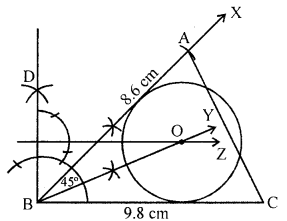(b) The marks obtained by T20 students in a Mathematics test are given below :

 Marks No. of students Marks No. of students 0-10 5 50-60 18 10-20 9 60-70 11 20-30 16 70-80 6 30-40 22 80-90 4 40-50 26 90-100 3

Using the informations, given above, draw an ogive on a graph sheet. Take a suitable scale for your ogive. Use the ogive drawn to estimate :
(i) the median.
(ii) the number of students who obtained more than 75% marks in the test.
(iii) the number of students who did not pass in the test if the pass percentage was 40.
Solution:

 Marks No. of students (f) c.f 0-10 5 5 10-20 9 14 20-30 16 30 30-40 22 52 40-50 26 78 50-60 18 96 60-70 11 107 70-80 6 113 80-90 4 117 90-100 3 120

Plot the points (10, 5), (20, 14), (30, 30), (40, 52), (50, 78), (60, 96), (70, 107), (80, 113), (90, 117), (100, 120) on the graph and join them free hand. We get an ogive as shown.Total students =120
Median : From = $$\frac{120}{2}$$ = 60
y-axis draw a line parallel to x-axis which meets the curve at B. From B, draw perpendicular on x- axis meeting it at C which is 43.
∴ Median = 43
(ii) No. of students getting more then 75% marks = 120 – 110= 10 students.
(iii) Number of students who did not pass the test (passing percentage = 40%) = 52

Question 11.
(a) Find the co-ordinates of the circumcentre of the triangle whose vertices are (3, 0), (-1, -6) and (4, -1). Also, find its circumradius.
Solution:
The vertices of ∆ABC are A (3, 0), B (-1, -6), C (4, -1)
Let (x, y) be the co-ordinates of the circumcentre O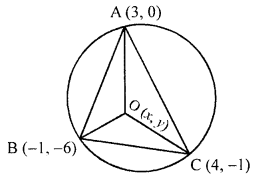∴ OA = OB = OC => OA2 – OB2 = OC2
OA= $$\sqrt{\left(x_{2}-x_{1}\right)^{2}+\left(y_{2}-y_{1}\right)^{2}}$$
OA2 = (x2 – x1)2 + (y2 – y1)2 = (x – 3)2 + (y – 0)2
= x2 – 6x + 9 + y2 = x2 + y2 – 6x + 9
Similarly OB2 = (x + 1)2 + (y + 6)2 = (x2 + 2x + 1 + y2 + 12y + 36 = x2 + y2 + 2x + 12y + 37
OC2 = (x – 4)2 + (y + 1)2 = x2 – 8x + 16 +y2 + 2y + 1
= x2 + y2 – 8x + 2y + 17
∵ OA2 = OB2
∴ x2 + y2 – 6x + 9 = x2 + y2 + 2x + 17ly + 37 ⇒ -8x – 12y = 28
⇒ 2x + 3y ⇒ – 7 ….(i)
and OB2 = OC2 ⇒ x2 +y2 + 2x + 12y + 37 = x2 + y2 – 8x + 2y + 17
∴ 10x +10y = -20 ⇒ x +y = -2 …(ii)
Solving (i) and (ii), y = -3, x = 1
∴ Co-ordinates of centre O are (1, -3)
Circumradius A = $$\sqrt{(3-1)^{2}+(0+3)^{2}}=\sqrt{2^{2}+3^{2}}=\sqrt{4+9}=\sqrt{13}$$ cm

(b) Use ruler and a pair of compasses to construct angle ABC = 75°. Mark a point D on BC, such that BD = 5 cm. Construct a circle to touch AB at B and also to pass through D.
Solution: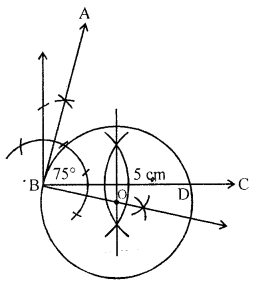Steps of construction :
(i) Construct ∠ABC = 75°
Using ruler and compass and take a point D such that BC = 5 cm.
(ii) Draw perpendicular bisector at BD.
(iii) Draw a perpendicular at B on AB which intersects the perpendicular bisector at O.
(iv) With centre O and radius D, draw a circle which passes through D and touches AB at B.
This is the required circle.

(c) ABC is a right-angled triangle with the right angle at vertex B. BD is the altitude through B. Given BD = 12 cm and AD – 9 cm.
(i) Calculate AB.
(ii) Name the triangle which are similar to triangle ADB (Proof not required).
(iii) Find AC.
Solution:
In right ∆ABC, ∠B = 90°
BD ⊥ AC
BD = 12 cm, AD = 9 cm
(i) ∴ AB2 = AD2 + BD2 (Pythagoras Theorm)
= 92 + 122 = 81 + 144 = 225
AB = $$\sqrt{225}$$ = 15 cm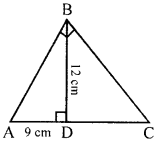(ii) ∆ABD ~ ∆ABC
and ∆ABD ~ ∆BCD
(iii) ∵ BD2 = AD × DC
∴ 122 = 9 × DC ⇒ $$\frac{144}{9}$$ =DC
⇒ DC = 16
∴ AC = AD + DC
= 9 + 16 = 25 cm